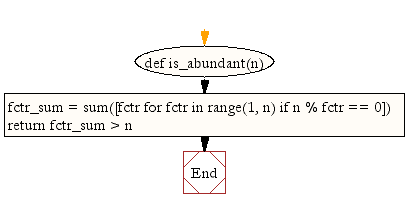﻿ Python Math: Find out, if the given number is abundant - w3resource# Python Math: Find out, if the given number is abundant

## Python Math: Exercise-13 with Solution

Write a Python program to find out, if the given number is abundant.
Note: In number theory, an abundant number or excessive number is a number for which the sum of its proper divisors is greater than the number itself. The integer 12 is the first abundant number. Its proper divisors are 1, 2, 3, 4 and 6 for a total of 16.

Sample Solution:-

Python Code:

``````def is_abundant(n):
fctr_sum = sum([fctr for fctr in range(1, n) if n % fctr == 0])
return fctr_sum > n
print(is_abundant(12))
print(is_abundant(13))
```
```

Sample Output:

```True
False
```

Pictorial Presentation:Flowchart:## Visualize Python code execution:

The following tool visualize what the computer is doing step-by-step as it executes the said program:

Python Code Editor:

Have another way to solve this solution? Contribute your code (and comments) through Disqus.

What is the difficulty level of this exercise?

Test your Programming skills with w3resource's quiz.

﻿

```>>> students = [{'name': 'John', 'score': 98}, {'name': 'Mike', 'score': 94}, {'name': 'Jennifer', 'score': 99}]